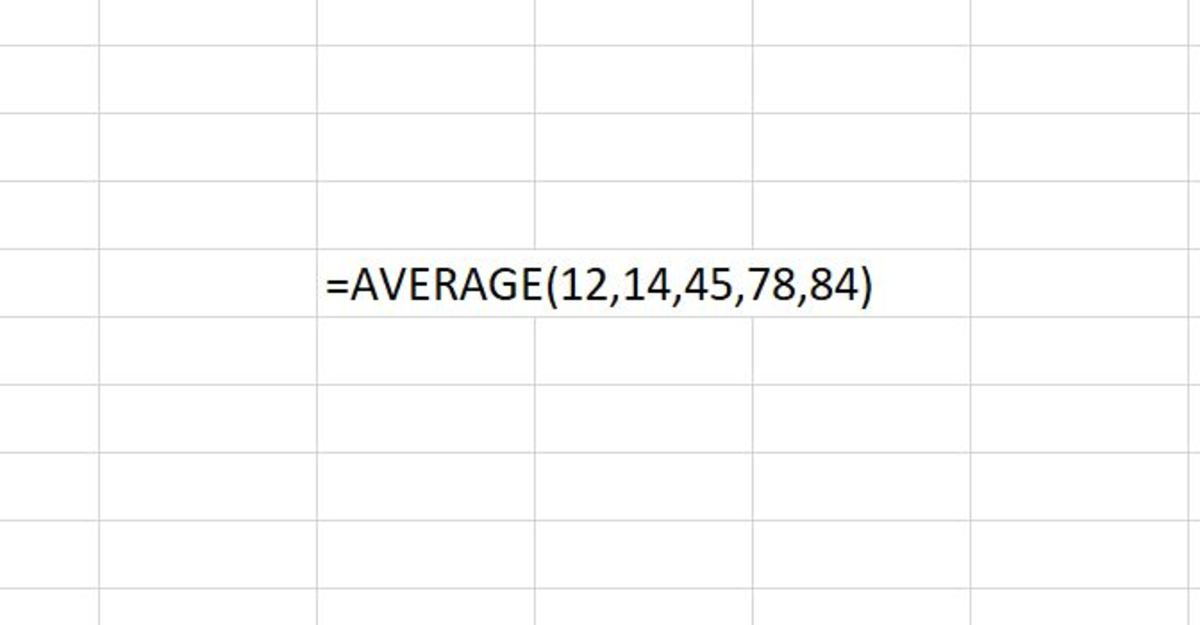# How to Use the AVERAGE Function in Excel

Joshua is a graduate student at the USF. He has interests in business technology, analytics, finance, and lean six sigma.An example of the AVERAGE function is shown in the above illustration. Created by Joshua Crowder

The AVERAGE function returns the average (mean or arithmetic mean) to a cell in Excel when used. The AVERAGE function adds a group of numbers and divides by how many numbers are in that group. For example, if one wanted to know the arithmetic mean of 4,5,6,7 they would add the numbers together and divide by 4.

(4+5+6+7)/4 = 5.5

The result of this calculation is the center of the group of numbers in a statistical distribution. This function is a measure of central tendency.

## The Syntax of the AVERAGE Function

The average function must follow the formats that are shown in the table below. Each format is further explained in the following sections.

## AVERAGE Function Syntax

DescriptionAverage Function Syntax

Average of a Referenced Range

=AVERAGE(A1:A5)

Average of a Referenced Range & Numbers

=AVERAGE(A1:A25,20)

Average of a Named Range

=AVERAGE(Range1)

Average of Cell References

=AVERAGE(A1,A2,A3,A4,A5)

Average of Numbers

=AVERAGE(12,14,45,78,84)

## Average of a Referenced Range

=AVERAGE(A1:A5)

In this situation, a cell reference range is used to find the arithmetic mean. This method is used when each number in a data set that needs to be averaged appears in its cell.

## Average of a Referenced Range & Numbers

=AVERAGE(A1:A25,20)

In this situation, a cell reference range and a number are used to find the arithmetic mean. This method is used when most numbers in a data set that needs to be averaged appear in a group of cells, and one or more numbers that don’t appear in cells may need to be added.

## Average of a Named Range

=AVERAGE(Range1)

In this situation, a named range is used to find the arithmetic mean of that range. This method is used when the data that is used to find the arithmetic mean has been previously named in a range.

## Average of Cell References

=AVERAGE(A1,A2,A3,A4,A5)

In this situation, cell references are used to find the arithmetic mean. This method may be used because the data needed to find the arithmetic mean is spread out throughout a worksheet.

## Average of Numbers

=AVERAGE(12,14,45,78,84)

Scroll to Continue

In this situation, numbers are used to find the arithmetic mean. This method is somewhat time-consuming since each number needs to be inputted into the function.

## Entering the AVERAGE Function Into a Worksheet

The average function needs to be inputted like a formula for it to be able to work. To manually add this formula to a cell, a cell needs to be clicked, and "=AVERAGE(" needs to be typed. After the open parenthesis, the data (numbers, range, named range, cell references) that are used to find the arithmetic mean need to be entered and separated by commas. This is then followed by a closing parenthesis. After the formula is created, the enter button can be pressed to return the average for the data.

The AVERAGE function can be inserted into a cell with the use of an insert tool for functions. To use this method a cell is first selected. Next, the formulas tab is selected and the "More Functions" button on the Excel ribbon needs to be selected. The statistical selection from the list is then chosen.

## Inserting the AVERAGE Function From the Formulas Tab

After the AVERAGE function argument window appears, a cell reference, arithmetic with cell references or numbers, needs to be added. There is enough room for 255 numbers or cell references. Next, the Ok button can be clicked to return the arithmetic mean into the cell.

## Other Considerations

Cells that are added to the AVERAGE function that will not be included in the calculation are cells, ranges, or named ranges that have logical values, empty cells, or text in them. Cells that contain a zero will be added to the calculation.

## How to Use The AVERAGE Function in Excel

Microsoft. (n.d.). AVERAGE function. Retrieved January 4, 2020, from https://support.office.com/en-us/article/average-function-047bac88-d466-426c-a32b-8f33eb960cf6.

Crowder, J. (2020, January 4). How to Use the AVERAGE Function in Excel. Retrieved January 4, 2020, from https://youtu.be/x_9YpHNlIvs.

## Learning More About Excel Functions

This content is accurate and true to the best of the author’s knowledge and is not meant to substitute for formal and individualized advice from a qualified professional.